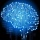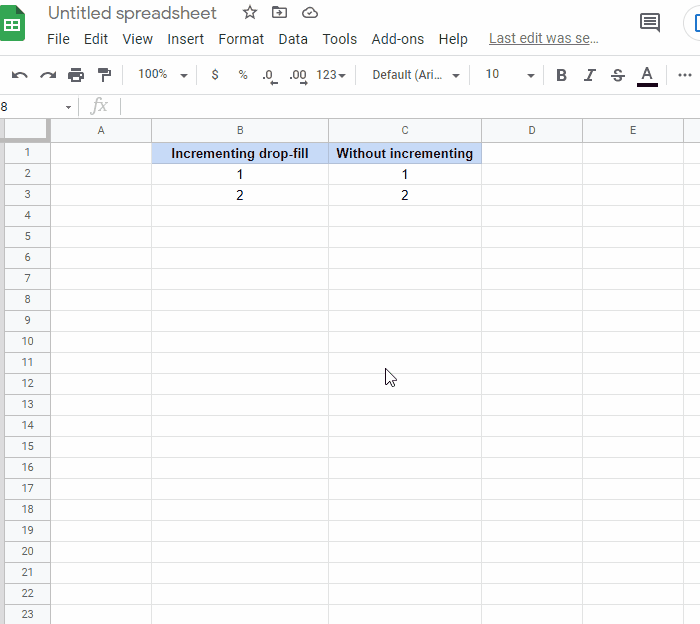Home
IT Knowledge
Inspiration
Languages
EN

# Google excel - prevent cells auto-changing when selected area is dragged

9 points
Created by:Root-ssh
121100

In this article, we would like to show you how to drag the values of the cells down without auto-changing them in Google Sheets.

Note:

In the example below, we use the `N` formula, which has a different application by default, but solves our problem.Alternatives:

``````=FIXED(1,0)
=FIXED(2,0)
=FIXED(3,0)``````
``````=TO_PURE_NUMBER(1)
=TO_PURE_NUMBER(2)
=TO_PURE_NUMBER(3)``````
``````=TO_TEXT(1)
=TO_TEXT(2)
=TO_TEXT(3)``````